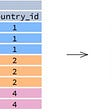# Lowest Common Ancestor of a Binary Tree — Day 104(Python)

Today we will be looking at a tree problem. It is a variation of the lowest common ancestor tree problem that we solved sometime back. The link to that problem can be found here. Let us look into today’s problem and how it is different from the previous question.

1644. Lowest Common Ancestor of a Binary Tree II

Given the `root` of a binary tree, return the lowest common ancestor (LCA) of two given nodes, `p` and `q`. If either node `p` or `q` does not exist in the tree, return `null`. All values of the nodes in the tree are unique.

According to the definition of LCA on Wikipedia: “The lowest common ancestor of two nodes `p` and `q` in a binary tree `T` is the lowest node that has both `p` and `q` as descendants (where we allow a node to be a descendant of itself)". A descendant of a node `x` is a node `y` that is on the path from node `x` to some leaf node.

Example 1:

`Input: root = [3,5,1,6,2,0,8,null,null,7,4], p = 5, q = 1Output: 3Explanation: The LCA of nodes 5 and 1 is 3.`

Example 2:

`Input: root = [3,5,1,6,2,0,8,null,null,7,4], p = 5, q = 4Output: 5Explanation: The LCA of nodes 5 and 4 is 5. A node can be a descendant of itself according to the definition of LCA.`

Example 3:

`Input: root = [3,5,1,6,2,0,8,null,null,7,4], p = 5, q = 10Output: nullExplanation: Node 10 does not exist in the tree, so return null.`

Constraints:

• The number of nodes in the tree is in the range `[1, 104]`.
• `-109 <= Node.val <= 109`
• All `Node.val` are unique.
• `p != q`

Follow up: Can you find the LCA traversing the tree, without checking nodes existence?

Now, the difference between this question and one that we solved earlier is, In this question, there is a possibility that the nodes may or may not exist. It means it is vital that we traverse through the entire tree.

One way to solve this problem is by having two boolean flags. Whenever we find our required node, we change the value of that boolean flag. This way we can know if we found both the nodes.

We will be using recursion to traverse through the tree.

1. Base condition -> We stop traversing tree if the the root is None.
2. Until then we keep moving left and right until reaching the leaf node. If the root is equal to any of the required node, we change the value in the boolean flag and return root.
3. If both the flags are true, then we return the value of root, else we return None.
`class Solution:    def lowestCommonAncestor(self, root: 'TreeNode', p: 'TreeNode', q: 'TreeNode') -> 'TreeNode':        self.p_found = False        self.q_found = False                def dfs(root, p, q):            if root == None:                return root            left = dfs(root.left, p, q)            right = dfs(root.right, p, q)            if root == p:                self.p_found = True                return root            if root == q:                self.q_found = True                return root            if left and right:                return root            return left or right        ans = dfs(root, p, q)        if(self.p_found == False or self.q_found == False):            return None        return ans`

Complexity analysis.

Time Complexity

We need to traverse the entire graph to find the nodes in the graph. Hence the time complexity is O(N).

Space Complexity

We are recursively traversing through the graph to find the nodes. We need to traverse the entire graph to find the nodes in the graph, and recursion is internally stored as a stack. Therefore the space complexity is O(N).

--

--

--

## More from Annamariya Tharayil

Software Engineer. Find me @ www.linkedin.com/in/annamariya-jt

Love podcasts or audiobooks? Learn on the go with our new app.

## How To Build Your Own API Using PHP## Distro Hopping, Manjaro XFCE 17.1 Edition## SQL Cheat Sheet -1-## Implement JsonResponse in Django API for JSON data## My Experience at Outbox EDU.## 2021 Top Software Development Trends and Benefits## Annamariya Tharayil

Software Engineer. Find me @ www.linkedin.com/in/annamariya-jt

## All Elements in Two Binary Search Trees — Day 108(Python)## Python Essentials Part 1 — args and kwargs in functions